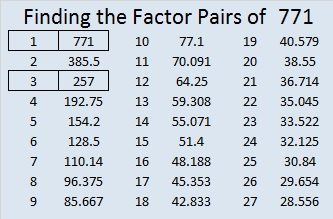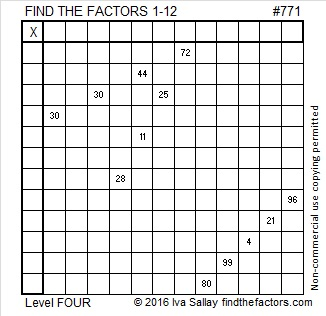# A regular 771-sided polygon can be constructed with a straightedge and compass.

Happy pi day to lovers of mathematics who happen to write their dates mm/dd/yyyy or mm/dd/yy (even for only one day a year)!

Lots will be written by others about pi today, but I’m going to write about the number 771 instead.

• 771 is a composite number.
• Prime factorization: 771 = 3 x 257
• The exponents in the prime factorization are 1 and 1. Adding one to each and multiplying we get (1 + 1)(1 + 1) = 2 x 2 = 4. Therefore 771 has exactly 4 factors.
• Factors of 771: 1, 3, 257, 771
• Factor pairs: 771 = 1 x 771 or 3 x 257
• 771 has no square factors that allow its square root to be simplified. √771 ≈ 27.76688675.The prime factors of 771 are 3 and 257, both of which are Fermat primes.  Wikipedia informs us that a regular 771-sided polygon can therefore be constructed using only a straightedge and a compass. Today’s puzzle looks a little like a compass and a straightedge:Print the puzzles or type the solution on this excel file: 12 Factors 2016-02-25

————————————-

Here is more about the number 771:

Like pi, √771 is irrational. The first few digits of √771 are 27.76688.

771 is the sum of three consecutive prime numbers: 251 + 257 + 263 = 771. One of those prime numbers is also a factor of 771.

Because 257 is one of its factors, 771 is the hypotenuse of a Pythagorean triple, and 96² + 765² = 771².

771 is also the sum of three squares four different ways. Notice that 11 and/or 19 appear in every one of those ways:

• 25² + 11² + 5² = 771
• 23² + 11² + 11² = 771
• 19² + 19² + 7² = 771
• 19² + 17² + 11² = 771

771 is a palindrome in FIVE different bases:

• 1100000011 BASE 2; note that 1(2^9) + 1(2^8) + 0(2^7) + 0(2^6) + 0(2^5) + 0(2^4) + 0(2^3) + 0(2^2) + 1(2^1) + 1(2^0) = 771.
• 30003 BASE 4; note that 3(4^4) + 0(4^3) + 0(4^2) + 0(4^1) + 3(4^0) = 771.
• 474 BASE 13; note that 4(169) + 7(13) + 4(1) = 771.
• 303 BASE 16; note that 3(256) + 0(16) + 3(1) = 771.
• 1D1 BASE 22 (D is 13 base 10); note that 1(22²) + 13(22) + 1(1) = 771.

————————————-## 2 thoughts on “A regular 771-sided polygon can be constructed with a straightedge and compass.”

1.travislj49

Do you have solutions to these puzzles in the spreadsheets?

•ivasallay

I do provide the solution to this puzzle in the spreadsheets, but at the bottom of this post there is a table that goes over a logical way to solve this particular puzzle.

This site uses Akismet to reduce spam. Learn how your comment data is processed.